# 23 Fun Subtraction Activities Kids and Teachers Will LoveFor some reason, subtraction is often a little trickier for students than addition. That’s why it’s good to have a whole collection of clever subtraction activities in your toolkit. These are some of our favorites for the schoolroom or to practice at home.

Anchor charts give kids something to refer back to as they work on new concepts and skills. We like this subtraction anchor chart for all the details it provides.

## 2. Read a subtraction book.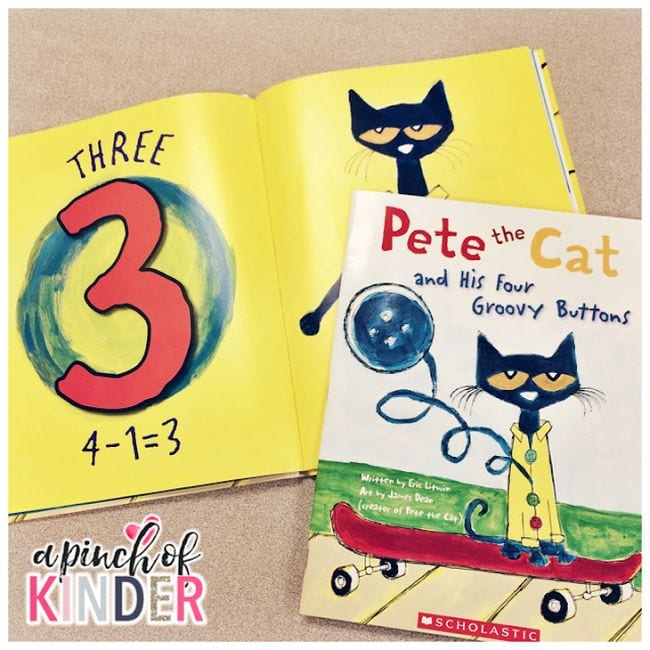Read-alouds are such a fun way to introduce new math concepts to kids. There are lots of great books to work into your subtraction activities, like our perennial favorite Pete the Cat and His Four Groovy Buttons

## 3. Count Pete’s buttons.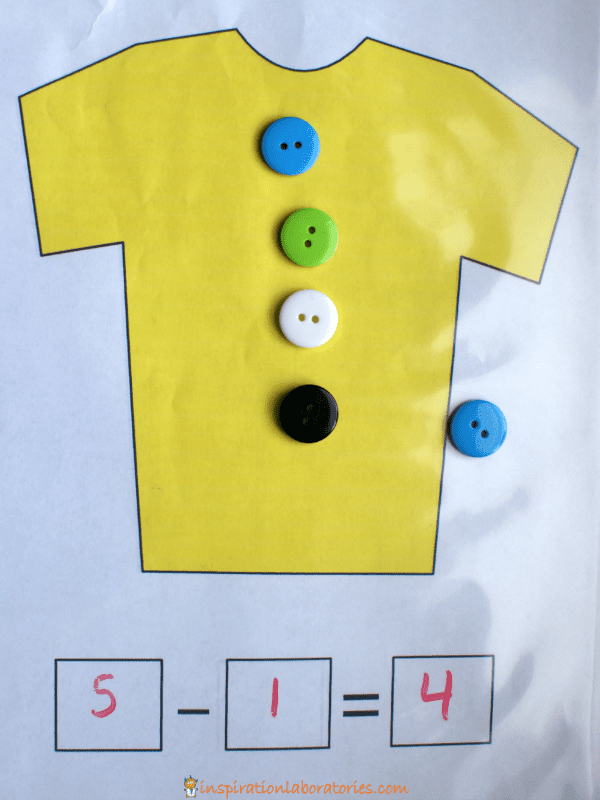If your students loved the book, then try this idea which incorporates real buttons into subtraction practice. So fun!

## 4. Climb the monkey tree.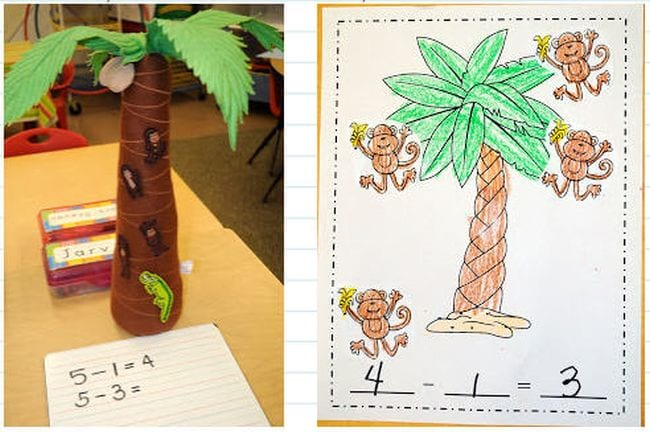Five Little Monkeys is another beloved book with math concepts worked right into the story. Build your own tree for monkeys to climb, or use a doll bed for jumping!

## 5. Introduce different strategies.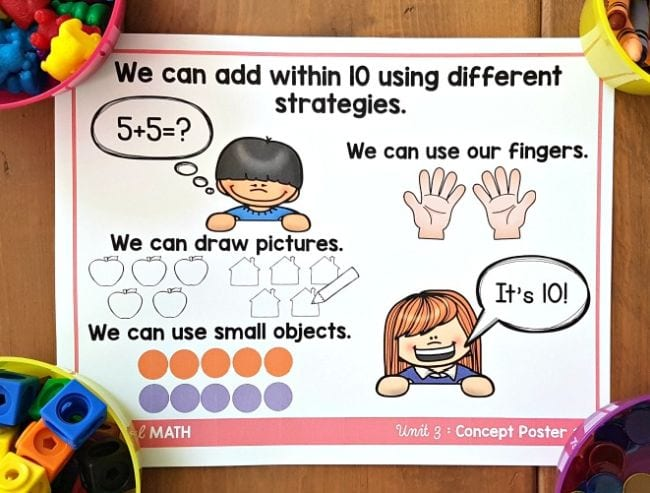Give kids plenty of strategies for understanding subtraction. They can use these when working on subtraction activities and exercises.

## 6. Smash some Play-Doh.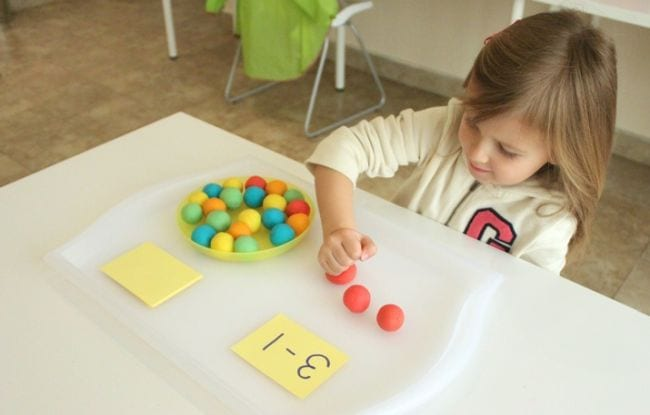For some reason, kids simply love to smash stuff! Roll balls of Play-Doh and let kids smoosh them as they subtract. (Find more ways to use Play-Doh for learning here.)

## 7. Whack-a-ball to subtract.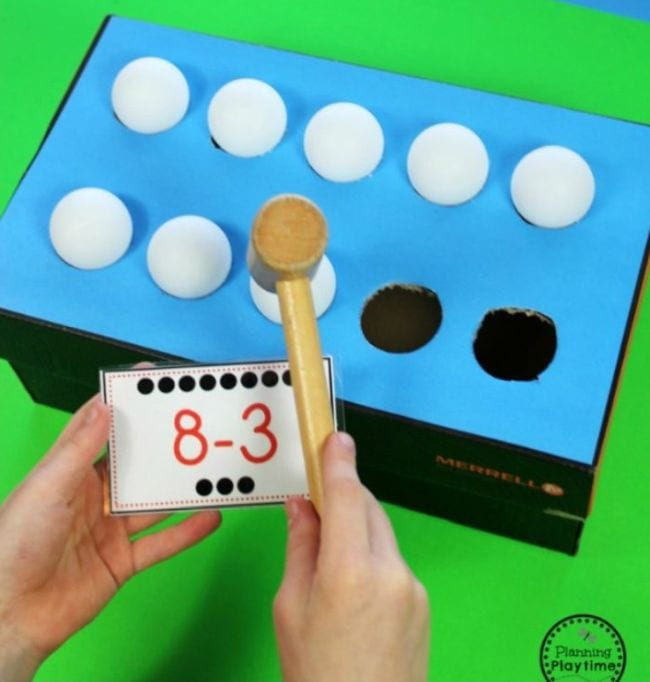Here’s another subtraction activity that lets kids burn off some energy! Make a 3-D ten frame by cutting holes in a shoebox. Rest ping pong balls on the holes, then whack them with a toy hammer to subtract.

## 8. Feed a hungry penguin.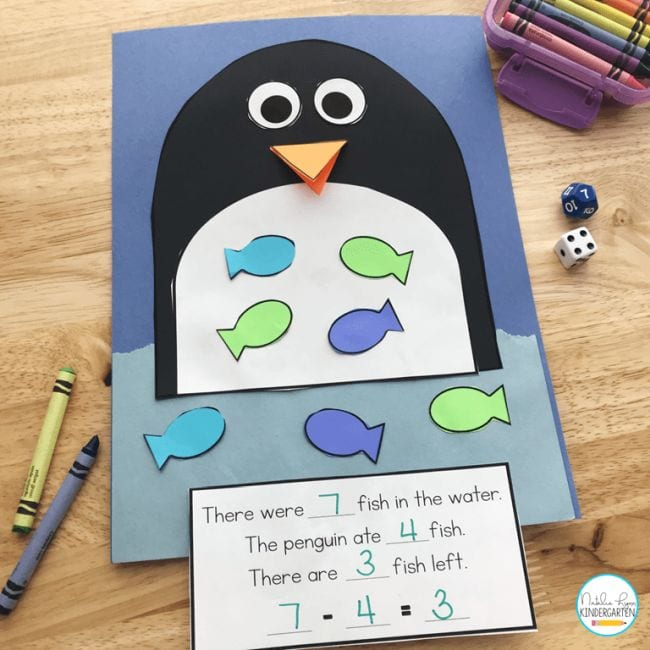This hungry penguin is here to help your students learn how to subtract! Feed him paper fishies and write out the equations.

## 9. Visualize the problem.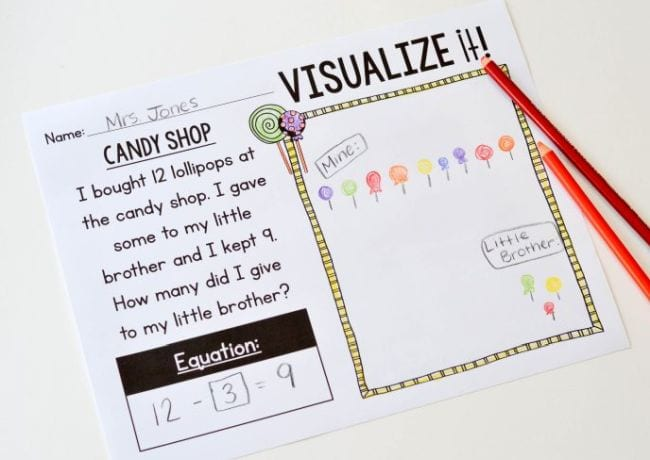Subtraction activities like story problems are easier when kids can visualize the action. Try drawing pictures or acting out the story to make the concept real.

## 10. Sort flash cards.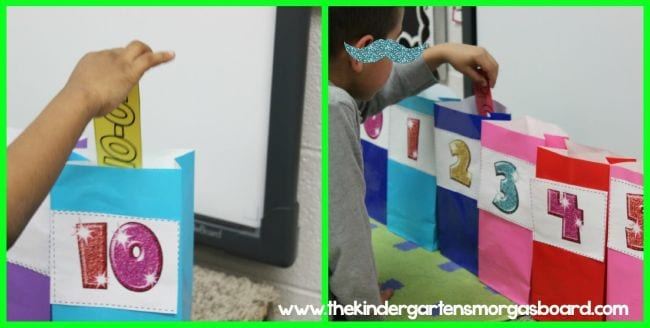Label a row of paper bags 1 to 10, then sort flashcards into the correct bags. Make it a timed exercise and have two teams race to see who’s the fastest (and most accurate)!

## 11. Bowl and subtract.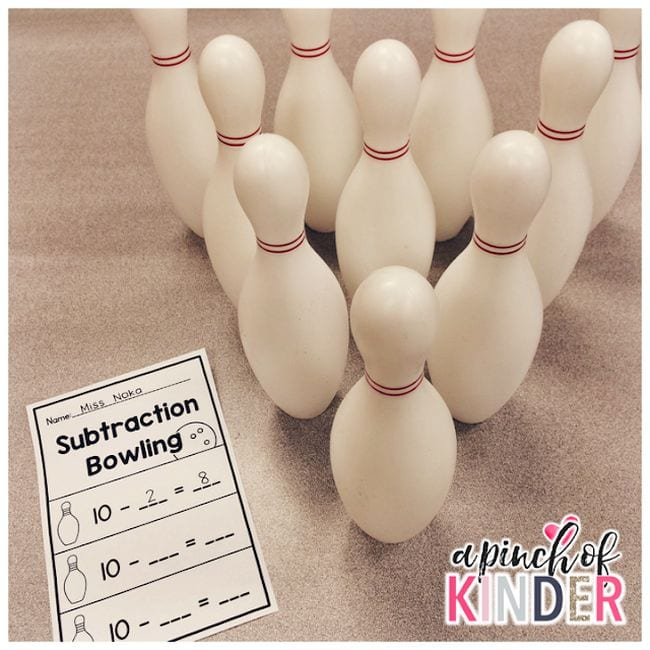This is one of the most popular subtraction activities out there. Kids bowl, knock down pins, and subtract them from the total. This is too much fun to be learning, right?

## 12. Have a LEGO subtraction race.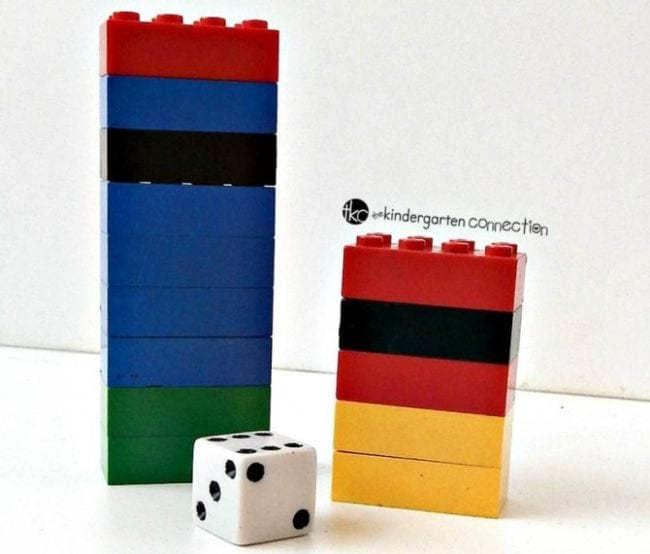Each person starts with a stack of LEGO bricks. Roll the die and take away the number shown, stating the equation out loud each time. First to zero wins!

## 13. Find the key to the lock.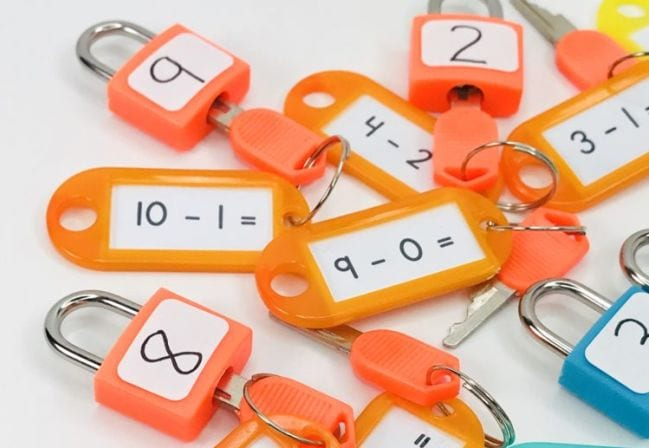Invest in a set of locks that you can use for all kinds of things, including subtraction activities. These 8-packs from Amazon come in a variety of colors and should last for years.

## 14. Use LEGO bricks for double-digits.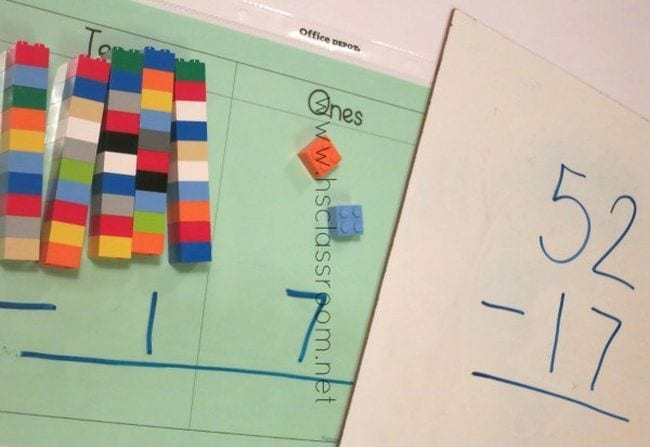Ready to talk about “borrowing” and double-digit math? Make the concept easier by using LEGO bricks.

## 15. Grab a hula hoop.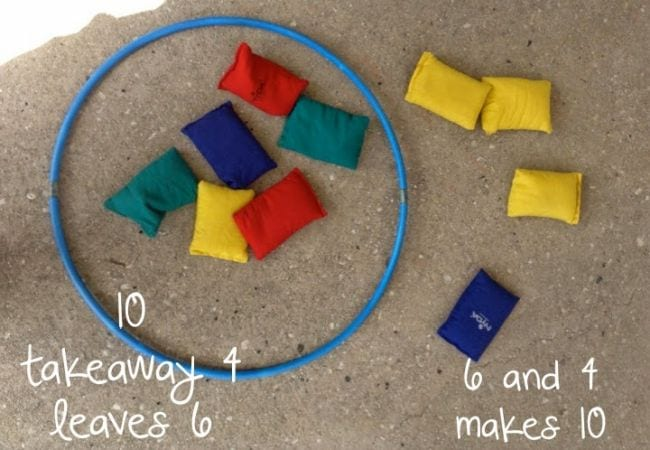Start by tossing bean bags and seeing how many you can get into the hoop. Then, turn the results into subtraction equations.

## 16. Ask “How many am I hiding?”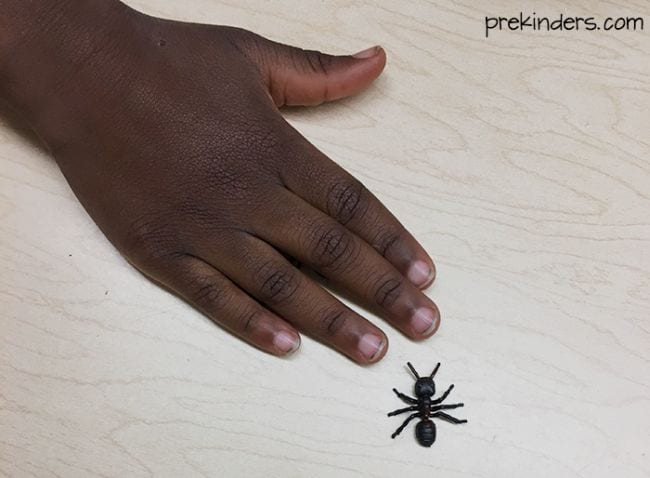Subtraction activities like this one are a fun way to introduce the idea to little learners. Start by laying a few small objects on a table. Next, cover some with your hand. Then, ask how many are left and how many you’re hiding. Simple, but effective.

## 17. Stack cups.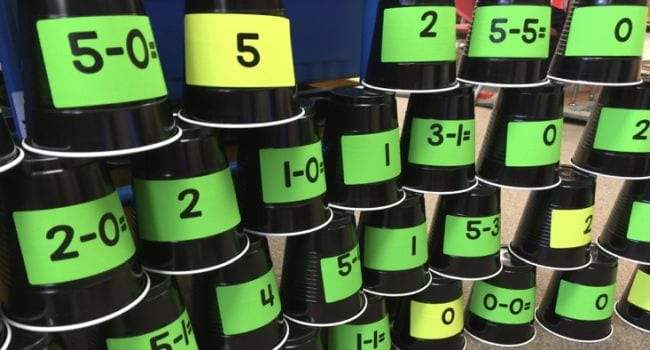Kids get such a kick out of stacking cups. Work subtraction practice into the mix by labeling the cups with equations and solutions, then challenge students to stack the cups to show the right answers.

## 18. Solve and play Bingo.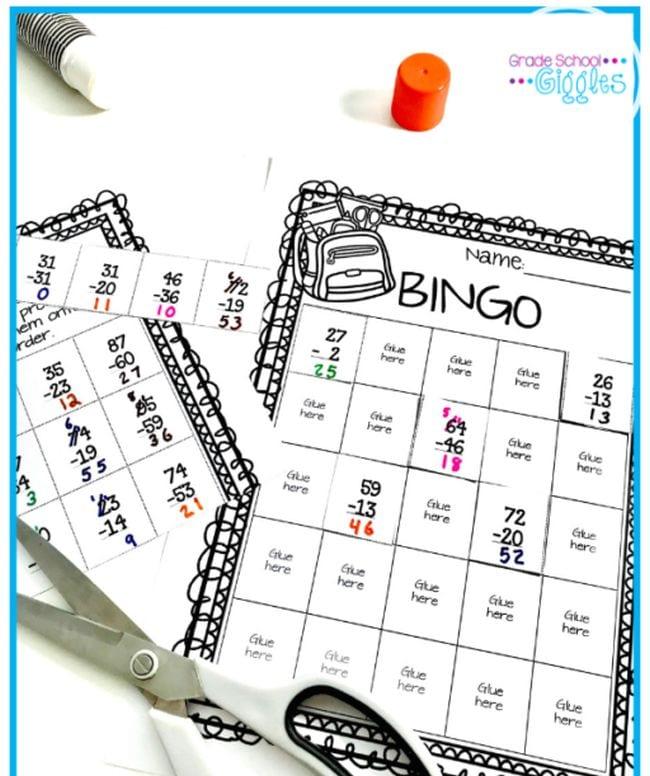First, kids work out the correct answers to the two-digit subtraction problems on this free printable. Then, they cut them out and paste them onto their Bingo board. Finally, play a game of Bingo together!

## 19. Toss some beanbags.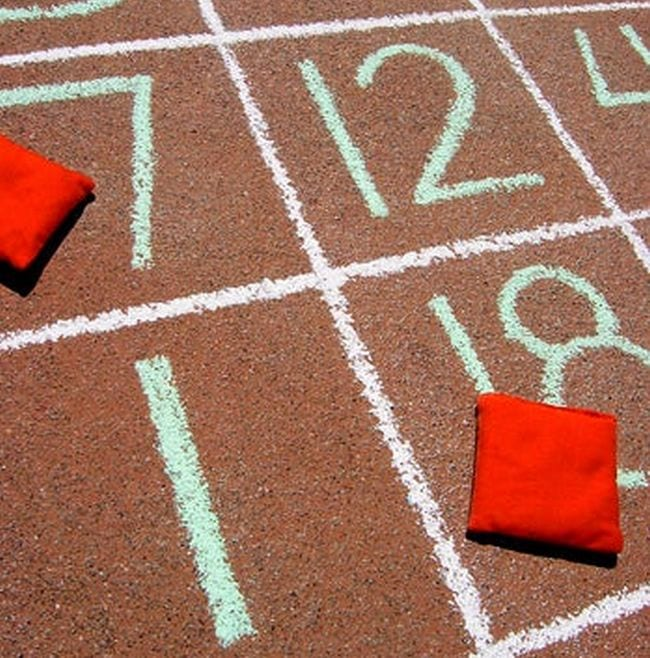Write a grid of randomized numbers on the sidewalk (or tape one off on your classroom floor). A student tosses two bean bags onto numbers, then makes a subtraction equation out of their results.

## 20. Use a zipper slider bag.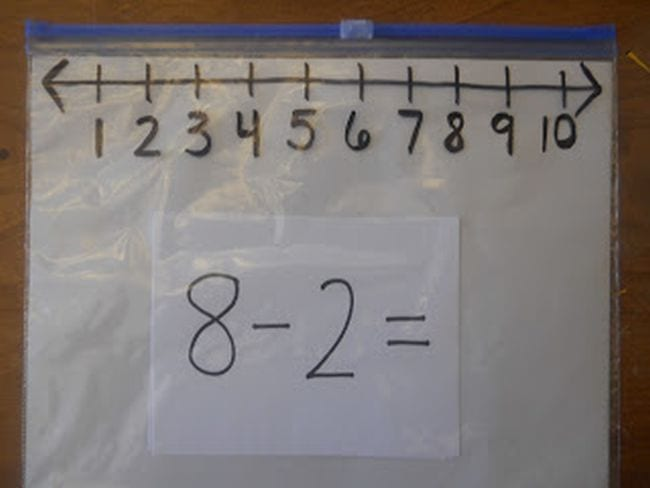A zipper bag number line? Genius! This clever idea is a useful tool for lots of subtraction activities. (Check out more number line ideas here.)

## 21. Slide beads on pipe cleaners.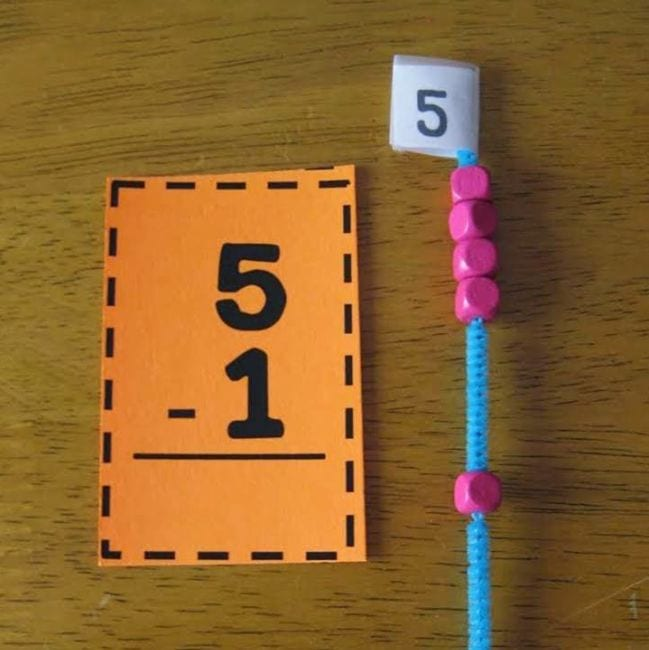Make some handy math manipulatives with pipe cleaners and beads. Slide the beads to see subtraction equations in action!

## 22. Build a wrecking ball.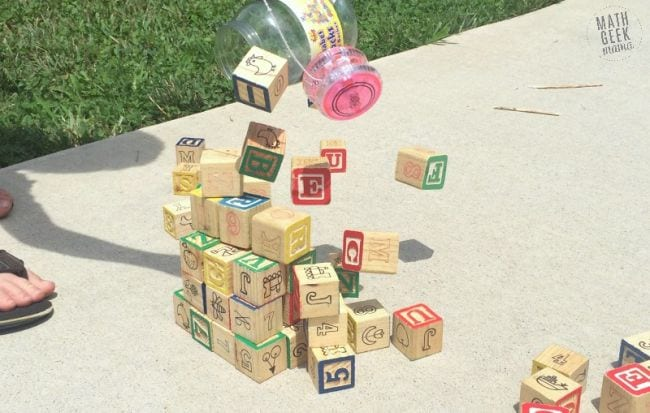One more chance to create a little mayhem! Similar to the bowling activity above, kids stack a number of blocks, then knock them down and see how many remain. Noisy, but fun!

## 23. Learn the subtraction rhymes.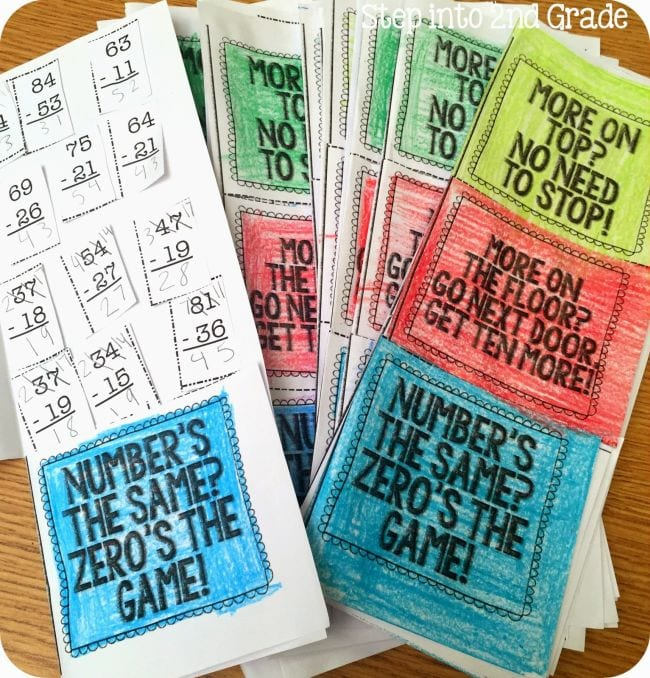These rhymes will aid kids working on more advanced subtraction activities, helping them remember when they need to “borrow” from the next place value column—and when they don’t.

Looking for more elementary math ideas? Check out these activities for teaching addition, multiplication, and division.

Plus, 60+ Awesome Websites for Teaching and Learning Math